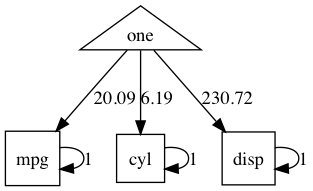# umx!

## “Staying on the (Wright) path”

`umxPath` is a core `umx` function. It facilitates path specification, streamlines scripts, and increases readability.

Let’s start using `umxPath` to specify a CFA model with a slight increase in complexity: two linked latent factors forced to be the same:We’ll also use `umxRAM` to give us automagical parameter labeling and good start values.

``````# 2. set up some handy lists for the built-in myFADataRaw data set
manifests = paste0("x", 1:3) # "x1" "x2" "x3"
latents = c("A", "B")

m1 <- umxRAM("m1", data = myFADataRaw,
# 1-headed paths from latent B to each of the manifests
umxPath("B", to = manifests),
# A slightly silly unit-covariance between latent 1 & 2
umxPath("A", with = "B", fixedAt = 1),
# free mean and variance for the manifests and standardized latents.
umxPath(v.m. = manifests),
umxPath(v1m0 = latents)
)
plot(m1, showFixed = TRUE)

``````

### Shortcuts

You just learned two shortcuts offered by `umxPath`: The “v1m0” and “v.m.” options are short for “variance @1 and mean @ zero” and `v.m.` is show for “variance free, mean free” respectively.

The intuition for `v.m.` is v for variance, m for means, and dot for “any value”

We could break this out into 4 lines if desired:

``````umxPath(means = manifests),
umxPath(var   = manifests),
umxPath(means = latents, fixedAt = 0),
umxPath(var   = latents, fixedAt = 1),
``````

The `umxPath` terms for connecting variables are shown below, along with their `mxPath` equivalents:

New Term Example mxPath Equivalent
var var = “X” from = “X”, arrows = 2
with “X”, with = “Y” from = “X”, to = “Y”, arrows = 2
cov cov = c(“X”,”Y”) from = “X”, to = “Y”, arrows = 2
means means = c(“X”,”Y”) from = “one”, to = c(“X”,”Y”)
v1m0 v1m0 = “X” requires 2 lines setting mean & var
v0m0 v0m0 = “Y” requires 2 lines setting mean & var
v.m. v.m. = “Y” requires 2 lines setting mean & var
defn defn = “A”, label=”data.Y” create a latent var@0 mean fixed, with data.var as label
unique.bivariate unique.bivariate = c(“X”,”Y”, “Z”) from = c(“X”,”Y”, “Z”), connection = “unique.bivariate”, arrows = 2
unique.pairs. unique.pairs = c(“X”,”Y”, “Z”) from = c(“X”,”Y”, “Z”), connection = “unique.pairs”, arrows = 2
Cholesky Cholesky = c(“X”,”Y”), to = c(“a”, “b”) a complex series of paths

### unique.bivariate

`unique.bivariate` is a time saver and error-preventer. It allows a 1-line specification of bivariate paths between all distinct pairs of variables.

So to create paths “A↔B”, “B↔C”, and “A↔C”, you can say just:

``````umxPath(unique.bivariate = c('A', 'B', 'C')
``````

and get back the equivalent of

``````umxPath("A", with  = "B")
umxPath("A", with  = "C")
umxPath("B", with  = "C")
``````

### Cholesky

Cholesky has a page of its own as this feature moves us into twin modeling.

### New words for setting values

The new words for setting values are shown below, along with their mxPath equivalents

New term mxPath Equivalent
fixedAt = 1 free = FALSE, values = 1
firstAt = 1 free = c(FALSE, TRUE, TRUE), values = c(1, NA, NA)
freeAt = .6 free = TRUE, values = .6

`firstAt` saves you laboriously counting out and keeping check of linked lists of free and value items. `freeAt` allows you to manually set a starting value.

### Connection types

umxPath still lets you do anything mxPath does, and one cool thing about mxPath is it has many connection types. I’ve exposed just unique.bivariate as a `umxPath` parameter, but the complete list of options is

1. “single”
2. “all.pairs”
3. “all.bivariate”
4. “unique.pairs”
5. “unique.bivariate”

Default value is “single”.

### Values and labels

You might have noticed that we can say things like

``````umxPath(c("A", "B", "C"), values = 1)
``````

Two things are happening here. First, the values field is being “recycled” for each of the three paths being created here, so the three paths will be started at 1. Second is the from field is being recycled into the to field. so:

``````umxPath(from  = "A")
``````

is the same as:

``````umxPath(from  = "A", to = "A")
``````

#### QuickLook: look often, run once

Tip: `plot`() is a great way to see what you are doing in a model as you build it: look often build once.

``````    plot(umxRAM("tim", umxPath(c("mpg", "cyl", "disp"), value=1), data=mtcars, run=F))
# hmmm single-headed arrows... should use "var = "
``````